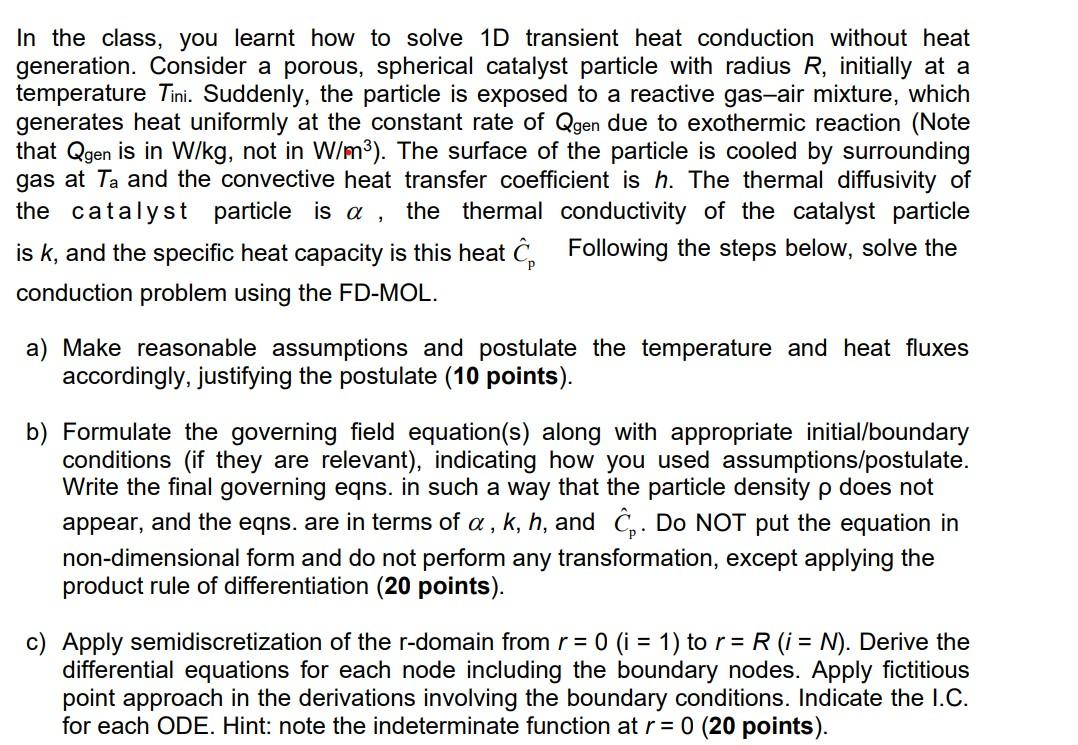# (Solved): Consider a porous, spherical catalyst particle with radius R, initially at a temperature Ti. Suddenl ...

Consider a porous, spherical catalyst particle with radius R, initially at a temperature Ti. Suddenly, the particle is exposed to a reactive gas–air mixture, which generates heat uniformly at the constant rate of Qgen due to exothermic reaction (Note that Qgen is in W/kg, not in W/m3). The surface of the particle is cooled by surrounding gas at Ta and the convective heat transfer coefficient is h. The thermal diffusivity of the catalyst particle is a , the thermal conductivity of the catalyst particles is k, and the specific heat capacity is this heat Cp, conduction problem using the FD-MOL. Following the steps below, solve the a) Make reasonable assumptions and postulate the temperature and heat fluxes accordingly, justifying the postulate b) Formulate the governing field equation(s) along with appropriate initial/boundary conditions (if they are relevant), indicating how you used assumptions/postulate. Write the final governing eqns. in such a way that the particle density ? does not appear, and the eqns. are in terms of a , k, h, and Cp .Do NOT put the equation in non-dimensional form and do not perform any transformation, except applying the product rule of differentiation c) Apply semidiscretization of the r-domain from r = 0 (i= 1) to r = R (i= N). Derive the differential equations for each node including the boundary nodes. Apply fictitious point approach in the derivations involving the boundary conditions. Indicate the I.C. for each ODE. Hint: note the indeterminate function at r = 0. PLEASE BREAKDOWN THE MATH AND STEPS AS CLEAR AND AS SIMPLE AS YOU CAN.In the class, you learnt how to solve transient heat conduction without heat generation. Consider a porous, spherical catalyst particle with radius , initially at a temperature Suddenly, the particle is exposed to a reactive gas-air mixture, which generates heat uniformly at the constant rate of due to exothermic reaction (Note that is in , not in ). The surface of the particle is cooled by surrounding gas at and the convective heat transfer coefficient is . The thermal diffusivity of the catalyst particle is , the thermal conductivity of the catalyst particle is , and the specific heat capacity is this heat Following the steps below, solve the conduction problem using the FD-MOL. a) Make reasonable assumptions and postulate the temperature and heat fluxes accordingly, justifying the postulate ( 10 points). b) Formulate the governing field equation(s) along with appropriate initial/boundary conditions (if they are relevant), indicating how you used assumptions/postulate. Write the final governing eqns. in such a way that the particle density does not appear, and the eqns. are in terms of , and . Do NOT put the equation in non-dimensional form and do not perform any transformation, except applying the product rule of differentiation ( 20 points). c) Apply semidiscretization of the -domain from to . Derive the differential equations for each node including the boundary nodes. Apply fictitious point approach in the derivations involving the boundary conditions. Indicate the I.C. for each ODE. Hint: note the indeterminate function at (20 points).

We have an Answer from Expert